# rERR package

fit Excess Relative Risk model

## Introduction

In radiation epidemiology, ERR models are used to analyze dose-response relationships for event rate data.

Usual approaches to the analysis of cohort and case control data often follow from risk-set sampling designs, where at each failure time a new risk set is defined, including the index case and all the controls that were at risk at that time. That kind of sampling designs are usually related to the Cox proportional hazards model,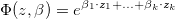where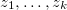is the vector of explanatory variables,

This model is available in most standard statistical packages but limited to log-linear model (except Epicure,(Preston et al., 1993)).

One model of particular interest, especially in radiation environmental and occupational epidemiology is the linear ERR model,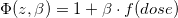where z is the vector of covariates.

Estimation of a dose-response trend under a linear relative rate model implies that for every 1-unit increase in the exposure metric, the rate of disease increases (or decreases) in an additive fashion.

The modification of the effect of exposure in linear relative rate models by a study covariate z can be assessed by including a log- linear subterm for the linear exposure, implying a model of the form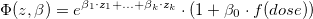whereare the covariates.

This package is an R impelementation of models that are rarely implemented outside Epicure: the Linear Excess Relative Risk model allowing time dependent covariates, time dependent adjustments, lagged exposures and stratification.

back

## Installing

• With compilation of source code: it requires Rtools installed in your computer

The package can be installed directly in RStudio from github:

install.packages("devtools") devtools::install_github("fbr600/rERR") * Without compilation:

Change the path to the download folder in the following code and run it in RStudio:

install.packages("path_to_downloads_folder/rERR.zip", repos = NULL, type = "win.binary") install.packages("devtools") devtools::install_deps(paste0(.libPaths(),"/rERR")) back

## Model Specification

• The ERR model is specified using a formula object in R

formula <- Surv(entry_time,exit_time,outcome) ~ lin(dose_cum) This is the formula for the model: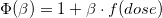where f(dose) is the cumulative dose of each subject up to the relative fail time.

• Also the covariates effect can be included in the model: formula <- Surv(entry_time,exit_time,outcome) ~ loglin(country) + lin(dose_cum) This is the formula of the model: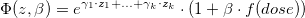whereare the different countries.

• Stratification in the risksets is done by using the strata() function: formula <- Surv(entry_time,exit_time,outcome) ~ loglin(country) + lin(dose_cum) + strata(sex) Is the same model as before, but in the risksets only the subjects of the same sex as the case are taken as in-risk-subjects.

Observation The data transformation required by the model computes the cumulative dose within the subject level over time, dose_cum, so this is a time-dependent variable, that is the cumulative dose recieved by each subject at every time.

This variable always can be used as a variable, or can be used to recompute new variables such as other polynomic terms, cateogrizations of dose…

back

## Latency period (lag) and exclusion

The latency period is the estimated time after a certain exposure in which the dose-response is not possible.

Analyzing the dose-response effect on a certain type of exposure, such as ionasing radiation, we want to exclude subjects that have a failure or disease before that period because of misleading on causation.

##### Risk Model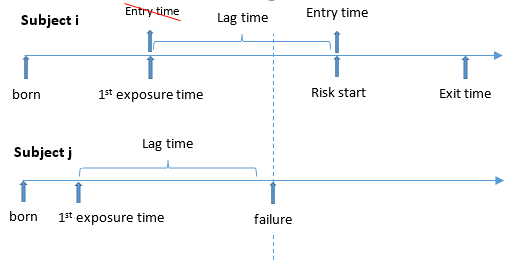So, imposing a latency period implies two actions: * Remove subjects that have a follow-up shorter than lag period time * Set the entry time to the cohort as 1st Exposure Time + lag , which is the moment in which a subject start being at risk

back

## Examples

Two different formats of data sets are allowed: * Event format data set (ef) - where each row represents an exposure event. * Wide format data set (wf) - where each row contain all the follow-up information of a subject, icluding times and doses of exposures

### Using an event format data set as input cohort

> library(rERR)
##### look at the data set
> head(cohort_ef,row.names=F)
id sex entry_age exit_age outcome  age     dose country
10   2      9.88 12.53114       0 7.88 15.80825      Be
14   1     11.09 12.35592       0 9.09 13.96742      UK
14   1     11.09 12.35592       0 9.17 13.81423      UK
15   1      9.55 12.33402       0 7.55  9.25016      Fr
16   1      8.48 12.29843       0 6.49 18.00776      Sp
16   1      8.48 12.29843       0 9.16 11.35068      Sp


##### set the formulas for two models

> formula1  <- Surv(entry_age,exit_age,outcome) ~ lin(dose_cum) + strata(sex)
> formula2  <- Surv(entry_age,exit_age,outcome) ~ loglin(factor(country)) + lin(dose_cum) + strata(sex)
##### fit the models
> fit1 <- f_fit_linERR_ef(formula1,data=cohort_ef,id_name="id",dose_name="dose",time_name="age",covars_names=c("sex"),lag=2,exclusion_done=T)
> fit2 <- f_fit_linERR_ef(formula2,data=cohort_ef,id_name="id",dose_name="dose",time_name="age",covars_names=c("sex","country"),lag=2,exclusion_done=T)
##### summary of fit1
> summary(fit1)
Formula:
Surv(entry_age, exit_age, outcome) ~ lin(dose_cum) + strata(sex)

Linear Parameter Summary Table:
coef   se(coef)         z  Pr(>|z|)
dose_cum 0.02401129 0.04400845 0.5456063 0.5853366

AIC:  313.8759
Deviance:  311.8759
Number of risk sets:  18 
##### summary of fit2
> summary(fit2)
Formula:
Surv(entry_age, exit_age, outcome) ~ loglin(factor(country)) +
lin(dose_cum) + strata(sex)

Linear Parameter Summary Table:
coef   se(coef)         z  Pr(>|z|)
dose_cum 0.02181835 0.04152847 0.5253829 0.5993171

Log Linear Parameter Summary Table:
coef exp(coef)  se(coef)          z  Pr(>|z|)
country_Fr 0.03444314  1.035043 1.0003142 0.03443232 0.9725324
country_Ge 0.70025564  2.014268 0.8660814 0.80853332 0.4187836
country_It 0.70146822  2.016712 0.8661484 0.80987072 0.4180145
country_Sp 0.04522023  1.046258 1.0001470 0.04521359 0.9639371
country_UK 0.69520396  2.004118 0.8661149 0.80266945 0.4221658

AIC:  321.9591
Deviance:  309.9591
Number of risk sets:  18
##### confidence intervals for fit1 parameters
> confint(fit1)
Confidence intervals:

Linear Parameter - Likelihood ratio test ci:
coef   lower .95 upper .95
dose_cum 0.02401129 -0.01109805 0.8468688
##### confidence intervals for fit2 parameters
> confint(fit2)
Confidence intervals:

Linear Parameter - Likelihood ratio test ci:
coef   lower .95 upper .95
dose_cum 0.02181835 -0.01138425  0.774703

Log Linear Parameter - Wald ci:
coef exp(coef) lower .95 upper .95
country_Fr 0.03444314  1.035043 0.1457101  7.352371
country_Ge 0.70025564  2.014268 0.3688989 10.998335
country_It 0.70146822  2.016712 0.3692980 11.013126
country_Sp 0.04522023  1.046258 0.1473371  7.429602
country_UK 0.69520396  2.004118 0.3670159 10.943634
##### likelihood ratio test between nested and nesting models
> f_lrt(fit1,fit2)
$lrt  1.916819$lrt_pval
 0.3835023

back

### Using a wide format data set as input cohort

> library(rERR)
##### look at the data set
> head(cohort_wf)
id sex YearInit AgeAtEntry age_at_event outcome end_status ses number_of_ct    ctage1 ctage2 ... ctage35 dose1 dose2 ... dose35 country
1   1     1983   7.957093    18.957093       0          2   1            1  7.957093      0           0  10.5     0          0      It
2   1     1988  13.857040    18.857040       0          2   1            2 13.857040      0           0  10.4     0          0      Sp
4   1     2008  16.496089    19.496089       0          2   1            1 16.496089      0           0   3.8     0          0      It
12   1     2004   8.134463    17.134463       0          2   3            2  8.134463      0           0   7.0     0          0      Sp
13   1     2009   2.554935     6.554935       0          2   2            2  2.554935      0           0   3.6     0          0      UK
16   1     1996  16.968197    18.968197       0          2   3            1 16.968197      0           0   3.5     0          0      Fr
##### set the formulas for the models
> formula1  <- Surv(AgeAtEntry,age_at_event,outcome) ~ lin(dose_cum) + strata(sex)
> formula2  <- Surv(AgeAtEntry,age_at_event,outcome) ~ loglin(factor(country)) + lin(dose_cum) + strata(sex)
##### fit the models
> fit1 <- f_fit_linERR_wf(formula1,data=cohort_wf,id_name="id",doses=cohort_wf[,45:79],times=cohort_wf[,10:44],
covars=cohort_wf[,c("sex","country")],lag=2,exclusion_done = F)

> fit2 <- f_fit_linERR_wf(formula2,data=cohort_wf,id_name="id",doses=cohort_wf[,45:79],times=cohort_wf[,10:44],
covars=cohort_wf[,c("sex","country")],lag=2,exclusion_done = F)
##### summary of fit1
> summary(fit1)
Formula:
Surv(AgeAtEntry, age_at_event, outcome) ~ lin(dose_cum) + strata(sex)

Linear Parameter Summary Table:
coef   se(coef)        z  Pr(>|z|)
dose_cum 0.03882416 0.03977917 0.975992 0.3290684

AIC:  599.129
Deviance:  597.129
Number of risk sets:  34
##### summary of fit2
> summary(fit2)
Formula:
Surv(AgeAtEntry, age_at_event, outcome) ~ loglin(factor(country)) +
lin(dose_cum) + strata(sex)

Linear Parameter Summary Table:
coef   se(coef)         z  Pr(>|z|)
dose_cum 0.0402001 0.04089224 0.9830742 0.3255709

Log Linear Parameter Summary Table:
coef    exp(coef)    se(coef)           z  Pr(>|z|)
country_Fr -14.827568523 3.634702e-07 616.7428233 -0.02404174 0.9808193
country_Ge  -0.005785829 9.942309e-01   0.5345654 -0.01082343 0.9913643
country_It   0.312718515 1.367137e+00   0.4929068  0.63443745 0.5257954
country_Sp  -0.606014765 5.455206e-01   0.6268803 -0.96671535 0.3336864
country_UK  -0.159932110 8.522016e-01   0.5565602 -0.28735816 0.7738381

AIC:  593.5899
Deviance:  581.5899
Number of risk sets:  34 
##### confidence intervals for fit1 parameters
> confint(fit1)
Confidence intervals:

Linear Parameter - Likelihood ratio test ci:
coef    lower .95 upper .95
dose_cum 0.03882416 -0.003425317  0.278199
##### confidence intervals for fit2 parameters
> confint(fit2)
Confidence intervals:

Linear Parameter - Likelihood ratio test ci:
coef    lower .95 upper .95
dose_cum 0.0402001 -0.003126192 0.2904963

Log Linear Parameter - Wald ci:
coef    exp(coef) lower .95 upper .95
country_Fr -14.827568523 3.634702e-07 0.0000000       Inf
country_Ge  -0.005785829 9.942309e-01 0.3487100  2.834720
country_It   0.312718515 1.367137e+00 0.5202941  3.592319
country_Sp  -0.606014765 5.455206e-01 0.1596650  1.863857
country_UK  -0.159932110 8.522016e-01 0.2862843  2.536806
##### likelihood ratio test between nested and nesting models
> f_lrt(fit1,fit2)
$lrt  15.53904$lrt_pval
 0.0004224168

back

### Save time in multi analysis of the same data set

The process of fitting the model include an internal transformation of the data that can slow down the time of all process.

Then if we need to run several analysis with the same data set, then we can run just one time the transfomation and run several analysis on the transformed data set.

The process can be splitted in three blocs: * Do the exclusion * Transform de data * fit the model

f_fit_linERR_ef <- function (formula, data, id_name, dose_name, time_name, covars_names,lag,exclusion_done=F)
{
# exclude subjects with the follow-up shorter than lag (lattency period) time
if(!exclusion_done)
data           <- f_exclusion(formula,data,lag)

# this piece of code is encapsuled in the function: f_to_event_table_ef_all
{
form          <- f_parse_formula(formula)
entry_name    <- as.character(form$Surv$entry)
exit_name     <- as.character(form$Surv$exit)
outcome_name  <- as.character(form$Surv$outcome)
dt1           <- f_to_event_table_ef_v2(id = id_name, start = entry_name,
stop = exit_name, outcome = outcome_name, data = data,
doses = dose_name, times = time_name, covars = covars_names)
}

# this piece of code is encapsuled in the function: f_fit_linERR_all
{
dt2           <- f_to_model_data(formula, data=dt1, id_name, time_name)
n_lin_vars    <- attr(dt2, "n_lin_vars")
n_loglin_vars <- attr(dt2, "n_loglin_vars")
rsets         <- f_risksets(formula, data = dt2, lag, id_name, time_name)
fit           <- f_fit_linERR(formula, data = dt2, rsets, n_lin_vars,
n_loglin_vars, id_name, time_name)
return(fit)
}
}

Then if the exclusion and the data transfomration are common in two analysis, we do not have to do it twice:

# specify the models we want to run
> formula1       <- Surv(entry_age,exit_age,outcome) ~ lin(dose_cum) + strata(sex)
> formula2       <- Surv(entry_age,exit_age,outcome) ~ loglin(factor(country)) + lin(dose_cum) + strata(sex)

# we only need to set the variables:
> data           <- cohort_ef
> exclusion_done <- TRUE
> lag            <- 2
> id_name        <- "id"
> time_name      <- "age"
> dose_name      <- "dose"

# do the exclusion if needed
> if (!exclusion_done)
data <- f_exclusion(formula, data, lag)

# transform the data including all the covariates required in all analysis
> dt1            <- f_to_event_table_ef_all(formula=formula1,data=data,id_name,dose_name,
time_name,covars_names=c("sex","country"))

# fit the first model
> fit1          <- f_fit_linERR_all(formula1,data=dt1,id_name,time_name)

# fit the second model
> fit2          <- f_fit_linERR_all(formula2,data=dt1,id_name,time_name)

back

### Using Categorical Exposure

Often we want to know how the risk evolves through levels of exposure, to do this we can create a categorization of the dose and then put this categories into the model in the loglinear part.

To do this the whole process can be broken in three main steps: * Do the first data transformation * Compute the categories of exposure * Fit the model, so run the optimization

##### Do the first transformation

Set the formula for the transformation and for the further categorical model, take into account that dose_cat still not exist, later on will be created:

> formula <- Surv(entry_age,exit_age,outcome) ~ loglin(dose_cat)+strata(sex)

Then run the transormation part, > dt1 <- f_to_event_table_ef_all(formula,data = cohort_ef,id_name = "id",dose_name = "dose", time_name = "age",covars_names = c("sex","country"))

Look at the new data set:

> head(dt1)
# A tibble: 6 x 13
# Groups:   id_ 
id   sex entry_age exit_age outcome   age  dose country   id_  n_pe dose_num dose_cum   id1
<int> <int>     <dbl>    <dbl>   <dbl> <dbl> <dbl> <chr>   <int> <dbl>    <dbl>    <dbl> <int>
1    10     2      9.88     12.5       0  7.88 15.8  Be         10     1    15.8     15.8      1
2    10     2      9.88     12.5       0 12.5   0    Be         10     0     0       15.8      1
3    14     1     11.1      12.4       0  9.09 14.0  UK         14     1    14.0     14.0      2
4    14     1     11.1      12.4       0  9.17 13.8  UK         14     2    13.8     27.8      2
5    14     1     11.1      12.4       0 12.4   0    UK         14     0     0       27.8      2
6    15     1      9.55     12.3       0  7.55  9.25 Fr         15     1     9.25     9.25     3
##### Compute the categories of exposure

The variable that will be categorized is dose_cum which is the cumulative dose at time age. To decide what are the more appropiate cutoffs for the exposure levels usually basic descriptives are used:

> summary(dt1$dose_cum) > hist(log(dt1$dose_cum))

Observation More accurate cutoffs can be described if only look at the doses that will be involved in the model, so it would imply to just look at the doses of the subjects in the risksets at the relatives failtimes.

After see the distributions of several sets of cutoffs, select the choice:

> dt1$dose_cat <- cut(dt1$dose_cum, breaks = c(0,5,10,25,100,1000), right=F )
> plyr::count(dt1\$dose_cat)
x  freq
1       [0,5) 18455
2      [5,10) 26262
3     [10,25) 46838
4    [25,100) 37005
5 [100,1e+03)  7490

Fit the model

> fit <- f_fit_linERR_all(formula,data=dt1,id_name = "id",time_name = "age",lag = 2)
> summary(fit)
Formula:
Surv(entry_age, exit_age, outcome) ~ loglin(dose_cat) + strata(sex)

Log Linear Parameter Summary Table:
coef    exp(coef)    se(coef)            z  Pr(>|z|)
dose_cat_[5,10)      -17.3175712 3.013522e-08 8388.608701 -0.002064415 0.9983528
dose_cat_[10,25)       0.7851632 2.192765e+00    1.094160  0.717594248 0.4730075
dose_cat_[25,100)      1.6628654 5.274403e+00    1.073705  1.548717024 0.1214498
dose_cat_[100,1e+03) -14.0343748 8.034308e-07 2372.656914 -0.005915046 0.9952805

AIC:  305.9834
Deviance:  297.9834
Number of risk sets:  18

back

### Plot the partial log likelihood function

There is an option to display the shape of the log likelihood function:

# set the formulas for the models
formula1  <- Surv(entry_age,exit_age,outcome) ~ lin(dose_cum) + strata(sex)

# fit the model
fit1 <- f_fit_linERR_ef(formula1,data=cohort_ef,id_name="id",dose_name="dose",
time_name="age",covars_names=c("sex"),lag=2,exclusion_done=TRUE)

# plot the partial loglikelihood function
f_plot_linERR_ef(fit1,formula1,data=cohort_ef,id_name="id",dose_name="dose",
time_name="age",covars_names=c("sex"),lag=2,exclusion_done=TRUE)

And it appears the following plot: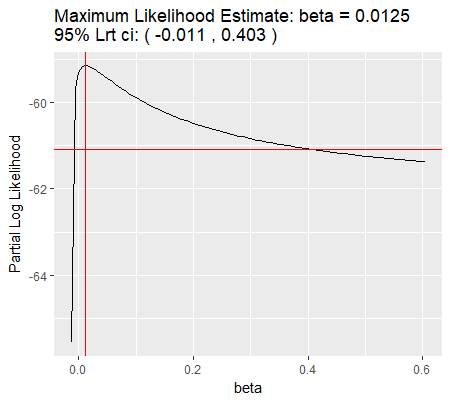back